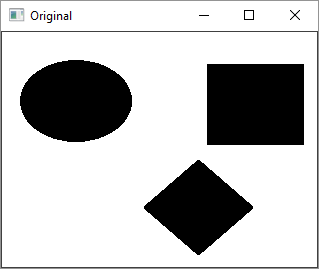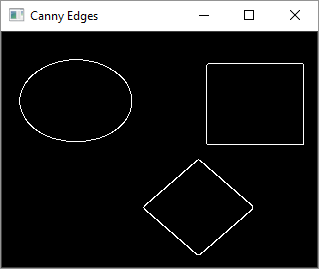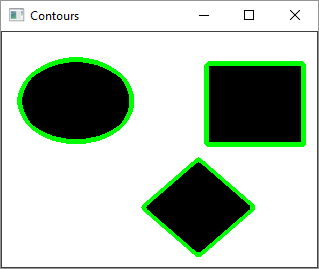# OpenCV Python - Image Contours

Contour is a curve joining all the continuous points along the boundary having the same color or intensity. The contours are very useful for shape analysis and object detection.

## Find Contour

Before finding contours, we should apply threshold or canny edge detection. Then, by using findContours() method, we can find the contours in the binary image.

The command for the usage of findContours()function is as follows −

cv.findContours(image, mode, method, contours)


### Parameters

The parameters of the findContours() function are as follows −

• image − Source, an 8-bit single-channel image.
• mode − Contour retrieval mode.
• method − Contour approximation method.

The mode parameter’s values are enumerated as follows −

• cv.RETR_EXTERNAL − Retrieves only the extreme outer contours.

• cv.RETR_LIST − Retrieves all of the contours without establishing any hierarchical relationships.

• cv.RETR_CCOMP − Retrieves all of the contours and organizes them into a twolevel hierarchy.

• cv.RETR_TREE − Retrieves all of the contours and reconstructs a full hierarchy of nested contours.

On the other hand, approximation method can be one from the following −

• cv.CHAIN_APPROX_NONE − Stores absolutely all the contour points.

• cv.CHAIN_APPROX_SIMPLE − Compresses horizontal, vertical, and diagonal segments and leaves only their end points.

## Draw Contour

After detecting the contour vectors, contours are drawn over the original image by using the cv.drawContours() function.

The command for the cv.drawContours() function is as follows −

cv.drawContours(image, contours, contourIdx, color)


### Parameters

The parameters of the drawContours() function are as follows −

• image − Destination image.
• contours − All the input contours. Each contour is stored as a point vector.
• contourIdx − Parameter indicating a contour to draw. If it is negative, all the contours are drawn.
• color − Color of the contours.

### Example

Following code is an example of drawing contours on an input image having three shapes filled with black colours.

In the first step, we obtain a gray image and then perform the canny edge detection.

On the resultant image, we then call findContours() function. Its result is a point vector. We then call the drawContours() function.

The complete code is as below −

import cv2
import numpy as np

cv2.imshow('Original', img)
gray = cv2.cvtColor(img, cv2.COLOR_BGR2GRAY)

canny = cv2.Canny(gray, 30, 200)

contours, hierarchy = cv2.findContours(canny,
cv2.RETR_EXTERNAL, cv2.CHAIN_APPROX_NONE)
print("Number of Contours = " ,len(contours))
cv2.imshow('Canny Edges', canny)

cv2.drawContours(img, contours, -1, (0, 255, 0), 3)

cv2.imshow('Contours', img)
cv2.waitKey(0)
cv2.destroyAllWindows()


### Output

The original image, after canny edge detection and one with contours drawn will be displayed in separate windows as shown here −After the canny edge detection, the image will be as follows −After the contours are drawn, the image will be as follows −